Posted by 陈树义 on 2021-06-17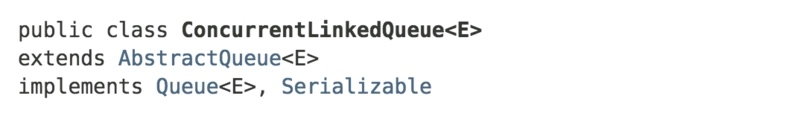## 队列结构

``````public class ConcurrentLinkedQueue<E> extends AbstractQueue<E>
implements Queue<E>, java.io.Serializable {

/**
* 队列头指针
*/
private transient volatile Node<E> head;

/**
* 队列尾指针.
*/
private transient volatile Node<E> tail;

// Unsafe mechanics

private static final sun.misc.Unsafe UNSAFE;
private static final long headOffset;
private static final long tailOffset;

static {
try {
UNSAFE = sun.misc.Unsafe.getUnsafe();
Class<?> k = ConcurrentLinkedQueue.class;
tailOffset = UNSAFE.objectFieldOffset (k.getDeclaredField("tail"));
} catch (Exception e) {
throw new Error(e);
}
}

/**
* 队列结点定义
*/
private static class Node<E> {
volatile E item;        // 元素值
volatile Node<E> next;  // 后驱指针

Node(E item) {
UNSAFE.putObject(this, itemOffset, item);
}

boolean casItem(E cmp, E val) {
return UNSAFE.compareAndSwapObject(this, itemOffset, cmp, val);
}

void lazySetNext(Node<E> val) {
UNSAFE.putOrderedObject(this, nextOffset, val);
}

boolean casNext(Node<E> cmp, Node<E> val) {
return UNSAFE.compareAndSwapObject(this, nextOffset, cmp, val);
}

// Unsafe mechanics

private static final sun.misc.Unsafe UNSAFE;
private static final long itemOffset;
private static final long nextOffset;

static {
try {
UNSAFE = sun.misc.Unsafe.getUnsafe();
Class<?> k = Node.class;
itemOffset = UNSAFE.objectFieldOffset(k.getDeclaredField("item"));
nextOffset = UNSAFE.objectFieldOffset(k.getDeclaredField("next"));
} catch (Exception e) {
throw new Error(e);
}
}
}

//...
}
``````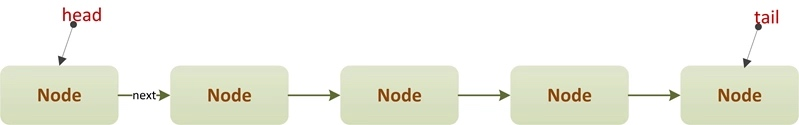## 构造器定义

``````/**
*/
head = tail = new Node<E>(null);
}
``````
``````/**
* 根据已有集合,构造队列
*/
public ConcurrentLinkedQueue(Collection<? extends E> c) {
Node<E> h = null, t = null;
for (E e : c) {
checkNotNull(e);
Node<E> newNode = new Node<E>(e);
if (h == null)
h = t = newNode;
else {
t.lazySetNext(newNode);
t = newNode;
}
}
if (h == null)
h = t = new Node<E>(null);
tail = t;
}
``````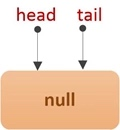## 入队操作

``````/**
* 入队一个元素.
*
* @throws NullPointerException 元素不能为null
*/
public boolean add(E e) {
return offer(e);
}
``````
``````/**
* 在队尾入队元素e, 直到成功
*/
public boolean offer(E e) {
checkNotNull(e);
final Node<E> newNode = new Node<E>(e);
for (Node<E> t = tail, p = t; ; ) {             // 自旋, 直到插入结点成功
Node<E> q = p.next;
if (q == null) {                            // CASE1: 正常情况下, 新结点直接插入到队尾
if (p.casNext(null, newNode)) {
// CAS竞争插入成功
if (p != t)                         // CAS竞争失败的线程会在下一次自旋中进入该逻辑
casTail(t, newNode);            // 重新设置队尾指针tail
return true;
}
// CAS竞争插入失败,则进入下一次自旋

} else if (p == q)                          // CASE2: 发生了出队操作
p = (t != (t = tail)) ? t : head;
else
// 将p重新指向队尾结点
p = (p != t && t != (t = tail)) ? t : q;
}
}
``````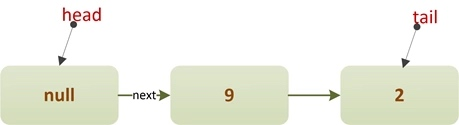``````if (q == null) {                            // CASE1: 正常情况下, 新结点直接插入到队尾
if (p.casNext(null, newNode)) {
// CAS竞争插入成功
if (p != t)                         // CAS竞争失败的线程会在下一次自旋中进入该逻辑
casTail(t, newNode);            // 重新设置队尾指针tail
return true;
}
// CAS竞争插入失败,则进入下一次自旋

}
``````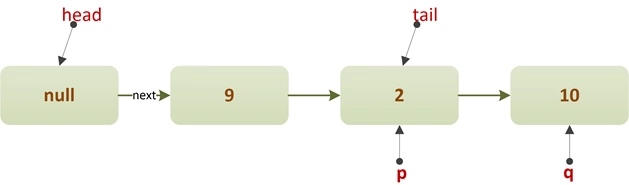``````else
// 将p重新指向队尾结点
p = (p != t && t != (t = tail)) ? t : q;
``````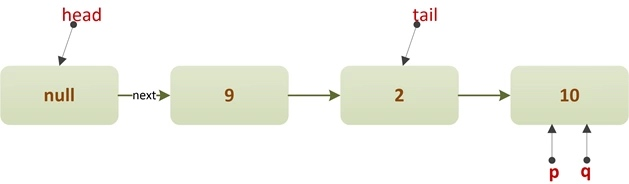``````if (q == null) {                            // CASE1: 正常情况下, 新结点直接插入到队尾
if (p.casNext(null, newNode)) {
// CAS竞争插入成功
if (p != t)                         // CAS竞争失败的线程会在下一次自旋中进入该逻辑
casTail(t, newNode);            // 重新设置队尾指针tail
return true;
}
// CAS竞争插入失败,则进入下一次自旋

}
``````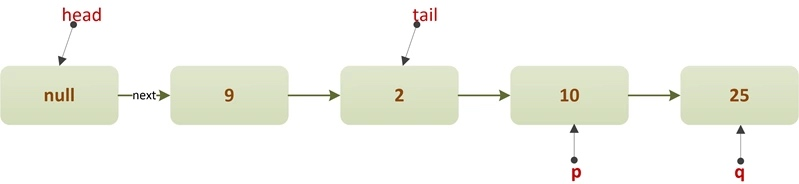``````casTail(t, newNode); // 重新设置队尾指针tail
``````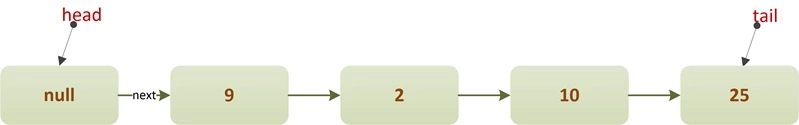``````else if (p == q)                          // CASE2: 发生了出队操作
p = (t != (t = tail)) ? t : head;
``````

## 出队操作

``````/**
* 在队首出队元素, 直到成功
*/
public E poll() {
for (; ; ) {
for (Node<E> h = head, p = h, q; ; ) {
E item = p.item;

if (item != null && p.casItem(item, null)) {    // CASE2: 队首是非哨兵结点(item!=null)
if (p != h) // hop two nodes at a time
updateHead(h, ((q = p.next) != null) ? q : p);
return item;
} else if ((q = p.next) == null) {      // CASE1: 队首是一个哨兵结点(item==null)
return null;
} else if (p == q)
else
p = q;
}
}
}
````````````else
p = q;
``````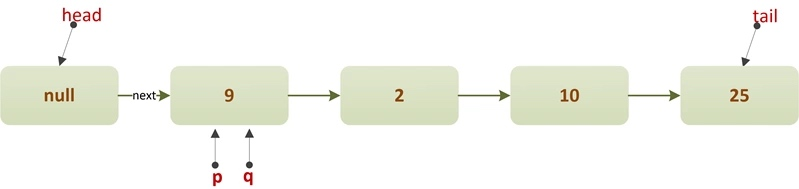``````if (item != null && p.casItem(item, null)) {    // CASE2: 队首是非哨兵结点(item!=null)
if (p != h) // hop two nodes at a time
updateHead(h, ((q = p.next) != null) ? q : p);
return item;
}
``````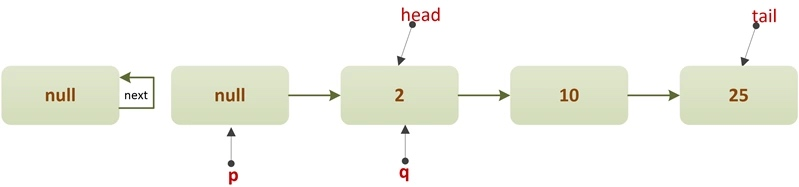CAS操作失败则会进入以下分支，并重新开始自旋：

``````else if (p == q)
``````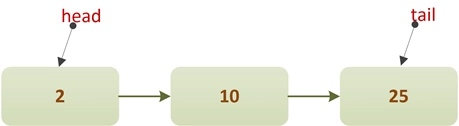``````if (item != null && p.casItem(item, null)) {    // CASE2: 队首是非哨兵结点(item!=null)
if (p != h) // hop two nodes at a time
updateHead(h, ((q = p.next) != null) ? q : p);
return item;
}
``````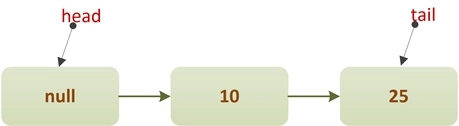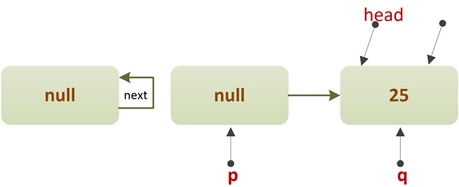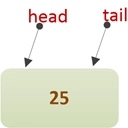`````` else if (p == q)                          // CASE2: 发生了出队操作
p = (t != (t = tail)) ? t : head;
``````

## 总结